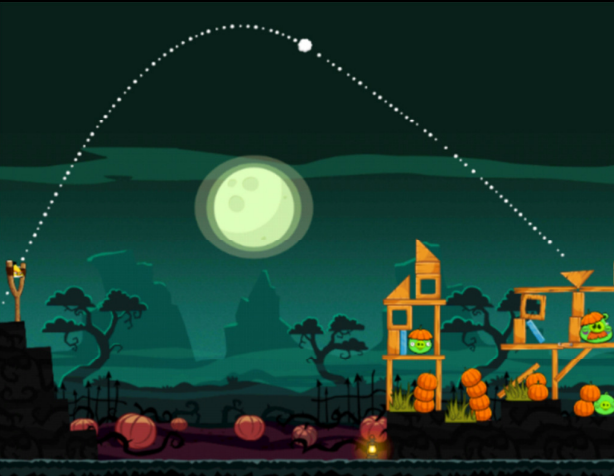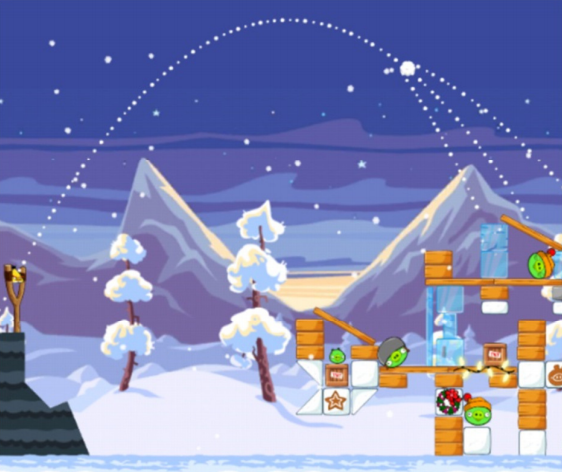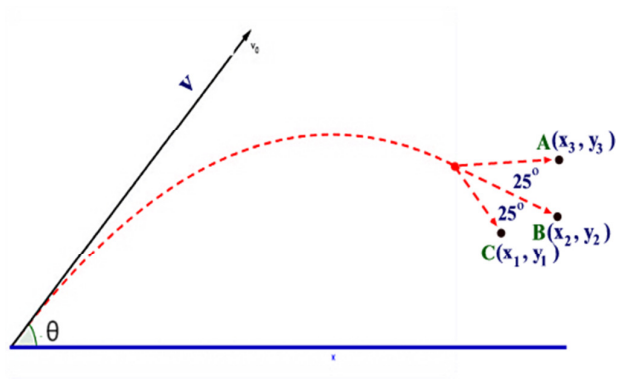시간 제한메모리 제한제출정답맞힌 사람정답 비율
3 초 256 MB365527.778%

## 문제

One of the most popular games for the Android, IOS world is Angry Birds which is developed by Rovio Entertainment Limited. Many different sorts of weapons are used in this game. Three of them have color red, yellow and blue. The red one has a conventional projectile path. The yellow one also has conventional projectile path but after throwing the weapon when the screen is touched again the yellow one continues to hold its line with a slight change in direction (The effect of g is almost nullified and shown in figure 1). On the other hand the blue one also has a projectile path when thrown but splits itself into three parts when the screen is touched again. The middle part continues in the original path of projectile while the other two goes slightly left or right (Shown in figure 2). In this problem we will introduce a new angry bird weapon which is a hybrid of these two (Yellow and Blue Weapon).Figure 1: Screen is touched when the yellow weapon reaches the location marked with a white circle. Figure 2: Screen is touched when the blue weapon reaches the location marked with a white circle. Figure 3: Paths of the new weapon

Our new weapon initially has the path of a projectile. But when screen is touched it splits itself into three parts and each of them continues in a straight line path. The middle part holds the direction of the projectile and continues in a straight-line. Other two parts make 25 degree angle with this direction on left and right side and continue in a straight line (As shown in figure 3). Finally those three parts hit three targets A, B and C at coordinates (x1,y1), (x2, y2) and (x3,y3).

Now in this problem you will be given the location of three targets and the weapon is always thrown from the origin (0,0). You will have to find the angle θ (expressed in degree) in which the weapon has to be thrown to hit the targets.

## 입력

First line of the input contains an integer T (T<=10000) which denotes how many test cases there are. Each of the next T lines contains a single test case. The description of each line is given below.

Each line contains six floating-point numbers x1, y1, x2, y2, x3 and y3 (500.0 <= x1, x2, x3 <= 10000.0) which denotes that the coordinate of three targets are (x1, y1), (x2, y2) and (x3, y3) respectively. The minimum distance between any two targets is 5 units.

## 출력

For each query produce one line of output. This line contains the serial of output followed by a floating-point number θ which denotes the angle (expressed in degree) in which the weapon needs to be thrown to make it able to hit all targets after exploding. This floating-point number should have six digits after the decimal point. If there is more than one solution, output the one that needs lesser initial speed to go along with it to hit the targets. Look at the output for sample input for details. You can assume that the weapons does not hit the target before splitting (even if it goes through the target) and there is no effect of wind or anything else on the weapon except the effect of g. But after splitting there is no effect of g on it as well. You can also assume that there will always be a solution (A valid value for θ). You must also assume that for a valid solution the throwing speed should be minimum 50 and maximum 5000 units and the throwing angle is in between 10 and 80 degree (Any solution that is not within this limit is also considered invalid). You should assume that g = 9.8 units/s2 .

## 예제 입력 1

2
6509.57475927 1176.42632622 6851.46336994 1279.22002747 7151.04630423 1589.26357354
4904.79101589 727.73576687 4782.48141525 1064.34391907 4699.50241228 426.80332837


## 예제 출력 1

Case 1: 49.329940
Case 2: 12.243520